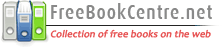# Free Differential Topology Books

 Abstract AlgebraLinear AlgebraCommutative AlgebraComplex AlgebraElliptic CurvesGeometric AlgebraGroups TheoryHigher AlgebraHomological AlgebraLie AlgebraDifferential AlgebraRings and FiledsGeometryAlgebraic GeometryDifferential GeometryFractalsRiemannian GeometryMathematical AnalysisComplex AnalysisFunctional AnalysisDifferential AnalysisFourier AnalysisHarmonic AnalysisNumerical AnalysisReal AnalysisTopologyAlgebraic TopologyDifferential TopologyGeometric TopologyK-theoryApplied MathematicsCalculusDifferential EquationsCombinatoricsDiscrete MathematicsGraph TheoryNumber TheoryProbability TheorySet TheoryTrigonometryCategory TheoryConstants & Numerical SequencesBasic MathematicsClassical AnalysisHistory of MathematicsArithmetic GeometryMathematical SeriesModern GeometryBasic AlgebraDifferential EquationsHome About Us Link to us Sitemap Contact Us Post Queries### This section contains free e-books and guides on Differential Topology, some of the resources in this section can be viewed online and some of them can be downloaded.

Differential Topology BooksIntroduction to Differential Topology by Uwe KaiserUwe KaiserPDF | 110 Pages | EnglishThis book gives a deeper account of basic ideas of differential topology than usual in introductory texts. Also many more examples of manifolds like matrix groups and Grassmannians are worked out in detail. Topics covered includes: Continuity, compactness and connectedness, Smooth manifolds and maps, Regular values and Sard’s theorem, Manifolds with boundary and orientations, Smooth homotopy and vector bundles, Intersection numbers, vector fields and Euler characteristic. Differential Algebraic TopologyMatthias KreckPDF | 168 Pages | EnglishThis book presents some basic concepts and results from algebraic topology. Topics covered includes: Smooth manifolds revisited, Stratifolds, Stratifolds with boundary: c-stratifolds, The Mayer-Vietoris sequence and homology groups of spheres, Brouwer’s fixed point theorem, separation and invariance of dimension, Integral homology and the mapping degree, A comparison theorem for homology theories and CW-complexes, Kunneth’s theorem, Singular cohomology and Poincare duality, Induced maps and the cohomology axioms, The Chern classes, Pontrjagin classes and applications to bordism, Constructions of stratifolds. Differential Topology by Bjorn Ian DundasBjorn Ian DundasPDF | 210 Pages | EnglishThis note covers the following topics: Smooth manifolds, The tangent space, Regular values, Vector bundles, Constructions on vector bundles and Integrability. Introduction To Differential TopologyJoel W. Robbin and Dietmar A. SalamonPDF | 207 Pages | EnglishThe first half of the book deals with degree theory, the Pontryagin construction, intersection theory, and Lefschetz numbers. The second half of the book is devoted to differential forms and deRham cohomology. Differential topology Lecture notes (PDF 20p)Sergei TabachnikovPDF | 20 Pages | EnglishThis note covers the following topics: Smooth manifolds and smooth maps, Tangent spaces and differentials , Regular and singular values , Manifolds with boundary, Immersions and embeddings , Degree mod 2 , Orientation of manifolds and Applications of degree. Combinatorial Differential Topology and Geometry (PDF 30p)Robin FormanPDF | 30 Pages | EnglishThis note explains how two standard techniques from the study of smooth manifolds, Morse theory and Bochner’s method, can be adapted to aid in the investigation of combinatorial spaces.Sponsored linksHome  |  About Us  |  Sitemap  |  Contact Us  |  Link to us  |  Post Your Queries |© Copyright 2015-2016. FreeBookCentre.net, All Rights Reserved. Privacy Policy  |  Terms & Conditions